• Call Now

1800-102-2727•

# Observer, Reference Frame, Inertial and Non Inertial Reference Frame, Practice Problems, FAQs

Let’s consider a situation, there is a bus moving on the road with some velocity. If I ask you what is the speed of the bus? Person standing on the ground would say it’s moving with some velocity. On the other hand a person seating in the bus will say it’s at rest. Also if there is another person riding on a bike he will have a different answer. Now if you analyse the situation you will understand that the answer depends on the observer. So to study the motion of a body you will first have to define the observer and frame of reference. We will study the reference frame and its types in this article.

• Observer
• Reference frame
• Types of reference frame
• Practice problems
• FAQs

## Observer

Observer is the one who observes the specified motion. Let’s take an example to understand. Consider a car moving towards the left with velocity 10 ms-1. There is person named A in the car and another person standing on the road named B. Now if we observe the motion of car with respect to observer A he will say that car is at rest so its velocity is 0 ms-1. On the other hand if we observe the motion of car with respect observer B, he will say that car is moving towards left with velocity 10 ms-1. Therefore we can say that the same motion can have different interpretations with respect to different observers. So in kinematics all the calculations are done with respect to the given observer. Before studying any motion we have to first define the observer.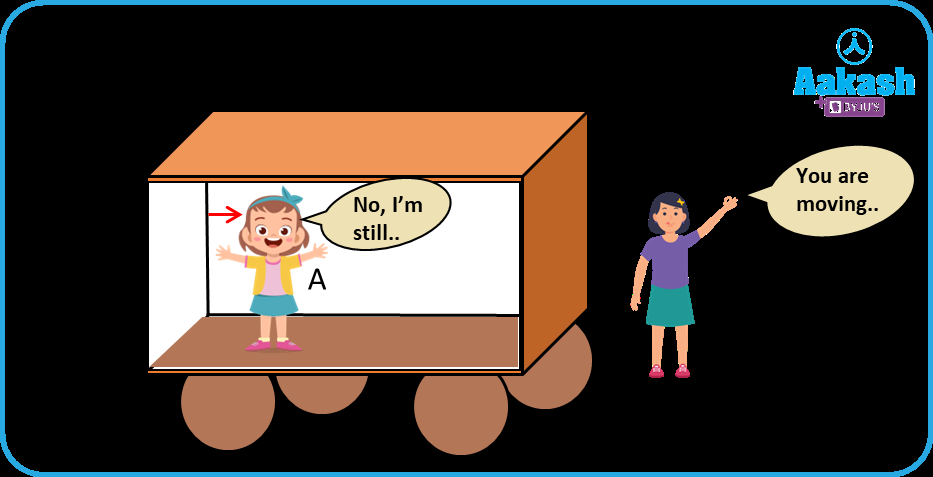## Reference frame

A frame in which an observer is situated and makes his observations is known as his/her 'frame of reference'.A frame of reference is defined as a set of coordinates which are needed to specify parameters like position or velocity of an object.  Anything that you observe, view, or measure will be compared to the reference point of the ground. We take the origin in the cartesian coordinate system as a reference point for the calculations.

Note: Velocity of A with respect to B is written as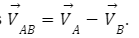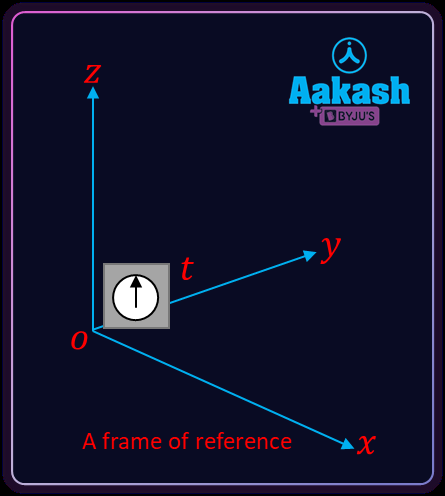In the above example for person A, a moving car is a frame of reference. For person B ground is the frame of reference.

## Types of reference frame

There are two types of reference frames.

1. Inertial Frame of Reference

• A frame of reference which is at rest or which is moving with a uniform velocity along a straight line is called an inertial frame of reference. A frame of reference is one in which Newton’s laws are obeyed is called an inertial frame of reference. It does not accelerate–unlike non-inertial frames.
• Inertial frame of reference is also known as Newtonian, Galilean or Unaccelerated frame of reference.
• Ideally no inertial frame of reference exists in the universe.Ideally no frame of reference in this universe is totally inertial. But we consider earth as an inertial frame of reference except while observing motion of planets. In this case the sun can be assumed as an inertial frame of reference.

Examples: 1) Observer at rest on the earth 2) A lift moving up/down with constant velocity.

2. Non-inertial Frame of Reference

• An accelerated frame of reference with respect to an inertial frame is called a Non-inertial frame of reference.
• Newton’s laws of motion are not valid in this frame of reference.

Newton’s laws of motion do not hold good in this frame of reference.

Examples: 1) Observer moving with some acceleration. Observer moving with non-zero acceleration. 2) Accelerated lift moving up/down.

Let’s take one interesting example to understand the non-inertial frame of reference. Consider a bus moving towards the right with some acceleration. As the bus is accelerating with respect to ground it is a non-inertial frame. Passenger sitting in the bus feels a backward push.Video Explanation: Frame of reference  (Time Stamp: 1.22.25 to 1.25.45)

## Practice Problems

Question.1 Give an example of an inertial frame of reference?

Answer: We consider earth as an inertial frame of reference. Any frame of reference which is not accelerated with respect to earth is an inertial reference frame. So a man standing on the ground is an example of an inertial reference frame.

Question.2 Given an example of a non-inertial frame of reference?

Answer: Frame reference which is accelerated with respect to inertial frame is called non inertial frame. An accelerated car is an example of an inertial reference frame.

Question.3 What is a reference point?

Answer: Origin is considered as a reference point for the calculation of physical quantities.

Question.4 Velocity of two persons A and B is given as 4i+5j+6k ms-1 and i+j+k ms-1  and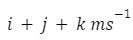respectively then find velocity of A as seen by observer B?

Answer: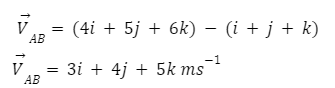FAQs

Question.1Define inertial frame of reference ?

Answer: A frame of reference is defined as the reference point .

Question.2 Define non-inertial frame of reference ?

Answer: An accelerated frame of reference with respect to an inertial frame is called a Non-inertial frame of reference.

Question.3 Are Newton’s laws of motion valid in a non inertial frame of reference?

Answer: No, Newton’s laws of motion are not valid in a non inertial frame of reference.

Question.4 What are the coordinates of origin in the cartesian plane?

Answer: (0,0,0) is the coordinates of origin in the cartesian plane.

Related Topics

 Pseudo Force- Inertial Vs Non-inertial Frame, Apparent Weight In Lift, Practice Problems, FAQs Newton’s First Law Of Motion, Inertia And Mass, Types Of Inertia, Practice Problems, FAQs Newton’s Second Law Of Motion- Its Mathematical Formulation, Derivation Of First Law Using Second Law, Impulse, Practice Problems, FAQs Newton Third Law Of Motion, Action Reaction Pair, Application Of Third Law, Third Law Contained In Second Law, Practice Problems, FAQsTalk to our expert
Resend OTP Timer =
By submitting up, I agree to receive all the Whatsapp communication on my registered number and Aakash terms and conditions and privacy policy